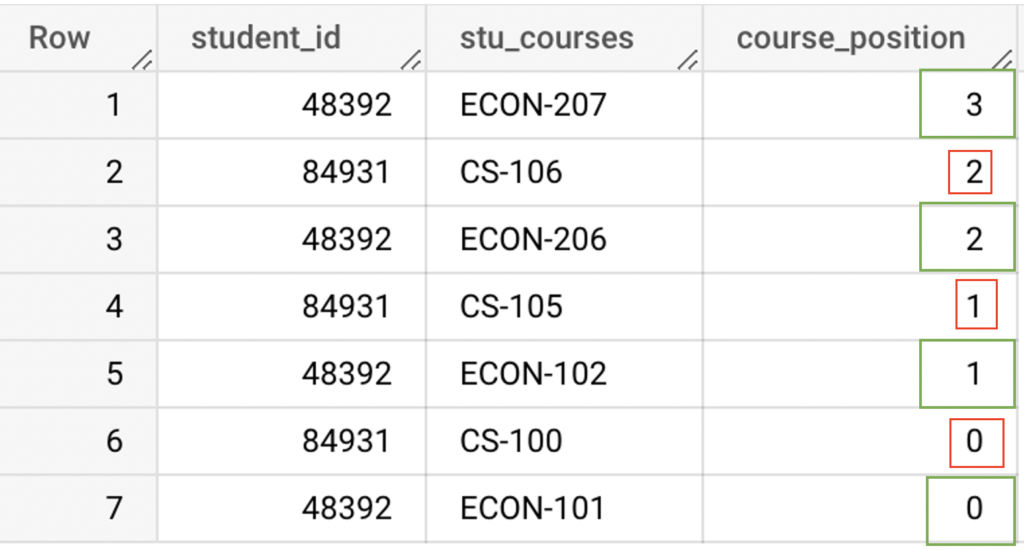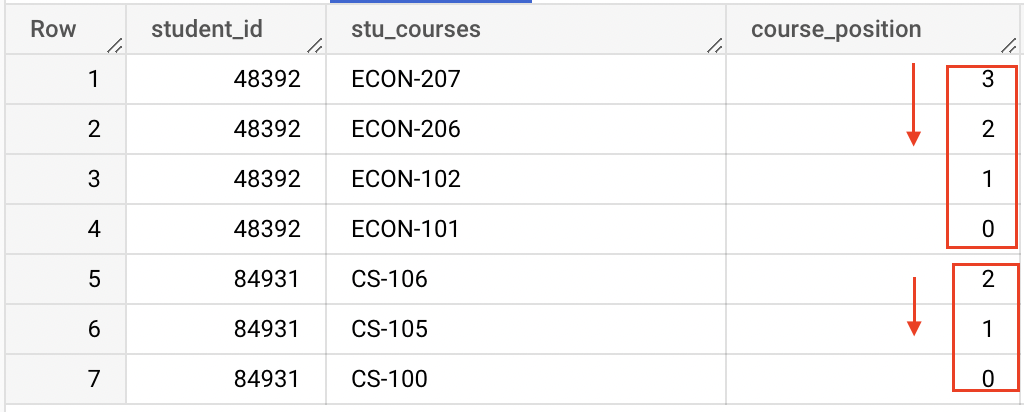# UNNEST function in BigQuery

UNNEST is an array function in BigQuery which is used to flatten out an array. It returns single row for each element in the array. Also it helps to fetch the element inside the array.

## Syntax for UNNEST function

As shown in the syntax, UNNEST function accepts only array data type values. To flatten the array columns/values, we need to use UNNEST after the FROM clause of an SELECT statement.

## Example

Let’s assume that, we have a table called as student_courses in BigQuery. It contains the course details of each student who are studying in the university. The table student_courses contains the following columns student_id, courses, semester, undergrad_program and academic_year.

The column courses is an ARRAY data type which has the set of course details. As shown below, the type of this column is STRING and mode is REPEATED. It means that we defined ARRAY<STRING> for the column courses.

Let’s look at the values inside the table student_courses in BigQuery. It contains course details of two students. For each student, set of course numbers are assigned in the column courses.

## Example 1 : Flatten the array

In this example, we will flatten the column courses using UNNEST function. To flatten the entire column of ARRAY values with existing values of other columns, we can use correlated cross join with UNNEST function.

As shown below, UNNEST flatten the ARRAY of courses into a set of rows. Then the CROSS JOIN joins these new set of rows with the single row from the existing table.

Also note that, CROSS JOIN with UNNEST is an optional. The same CROSS JOIN can be defined as a comma join.

As shown below, we got the same results using UNNEST. Here we didn’t mention any CROSS JOIN in the query.

## Example 2: UNNEST with OFFSET

The UNNEST function provides WITH OFFSET option to get the position of elements in the array. It returns additional column with the index/position of the element.

As shown below, we have flatten the array of courses with UNNEST and WITH OFFSET. For the offset, we have added the alias name as course_position.

• student_id #48392: It has 4 courses in the array. So it returned the offset value starting from 0 to 3.
• student_id #84931: It has 3 courses in the array. As a result, it returned the offset value starting from 0 to 2.

## Example 3: ORDER BY clause with OFFSET

Using ORDER BY clause, we can order the rows by their offset value. Let’s order the rows in descending order

As shown below, we have ordered the course_position in descending order.

• student_id #48392: It has 4 courses in the array. And, we ordered the offset value of array elements from 3 to 0.
• student_id #84931: It has 3 courses in the array. And, we ordered the offset value of array elements from 2 to 0.Flatten the array with OFFSET and ORDER BY clause in BigQuery

Let’s order the offset value with student_id. So that we can see the results more clearly.UNNEST WITH OFFSET and ORDER BY Clause in BigQuery

If we want to filter the results based on student_id, we can use the WHERE condition as below.

## Example 4: Aggregate function with UNNEST

Let’s do some analytics on the table student_courses. Consider that we want to find the number of courses taken by each student.

To do so, we can use COUNT function with GROUP BY clause. Since the courses are in ARRAY, we can use UNNEST function to get the single row for each element in the array.

As we shown below, the student with id #48392 has taken 4 courses and the other student with id #84931 has taken 3 courses.

## Example 5: UNNEST function with INNER JOIN

For this example, we have created one more table called as courses_info. It contains all the details about each course. This table has the following columns course_id, course_name and lecturer.

As we mentioned earlier, the table student_courses has a column courses which consist of array of course_id. Using that, we want to find the course name and lecturer of the course which is taken by the student.

For that, we can do the inner join on the tables student_courses & courses_info using course_id. Let’s break this into multiple steps as below

• UNNEST : Flatten the array of courses from the table student_courses.
• WITH clause: Create the temporary table from the results of previous step using WITH clause
• INNER JOIN: Inner join the temporary table and courses_info using below columns
• courses from table temporary table
• course_id from table courses_info

As shown below, we joined the student_courses and courses_info table and got the course details for each student.